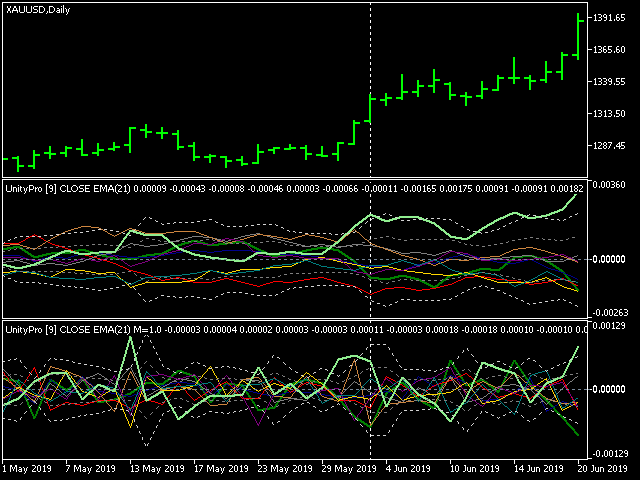Watch how to download trading robots for free
Interesting script?
So post a link to it -
let others appraise it
You liked the script? Try it in the MetaTrader 5 terminal# Unity Pro - indicator for MetaTrader 5

Views:
12058
Rating:
votes: 39
Published:
2019.08.30 12:37
Updated:
2019.08.31 16:04

This is an extended and improved version of cluster multi-asset indicator Unity. It shows synchronous changes in relative values of currencies, metals and optionally other assets.

The new version differs from the original indicator by slightly changed formula and added features.

The underlying algorithm can be described in the following way. Consider it on the minimalistic example with one currency pair (EURUSD) and gold (XAUUSD).

At every moment, the market state (that is current prices, or open prices of the bars) is expressed by obvious relations:

EUR / USD = EURUSD

XAU / USD = XAUUSD

where variables EUR, USD, XAU are pure "values" of the assets, and EURUSD, XAUUSD are constants (exchange rates, or quotes).

To find the variables, we supplement the system with another equation, limiting the sum of the variables to unity:

EUR + XAU + USD = 1

Hence the name of the indicator — Unity (Pro).

And here is the difference from Unity: while that used the sum of squares, this uses plain sum (no squares). As a result, if a user selects the mode AbsoluteValues (see below), any pair of lines of the indicator is related as corresponding exchange rate on every bar. For example, a value of EUR and a value of USD conforms to EURUSD (as a number). Please, note, this only gives proper ratio when moving averaging is off, that is PricePeriod is 1.

Simple substitution gives:

EURUSD * USD + XAUUSD * USD + USD = 1

Then USD can be found as:

USD = 1 / (1 + EURUSD + XAUUSD)

and all other variables can be calculated.

In general:

x0 = 1 / (1 + sum(C(xi, x0))), i = 1..n

xi = C(xi, x0) * x0, i = 1..n

where n — number of variables, C(xi,x0) — the quote of i-th pair including corresponding variables. Please note, that the number of variables is 1 more than instruments.

Elimination of squares and square roots improved indicator performance in comparison to Unity.

Since the coefficients C involved in the calculations are quotes that are usually very different, in the indicator they are additionally multiplied by contract's sizes: this gives more or less comparable values (at least, of one order). To see them "as is" in the indicator window there is an AbsoluteValues input parameter that should be set to true. By default, it is false, and the indicator calculates the increments of variables:

yi = xi0 / xi1 - 1,

where xi0 and xi1 are values on the last and the previous bars respectively.

Here is a screenshot for the cluster "EURUSD,GBPUSD,USDCHF,USDJPY,AUDUSD,USDCAD,NZDUSD,XAUUSD" in two instances of the indicator: with values and momentum (impulse):The lines of assets that make up work symbol of the current chart (in this case, XAU and USD) are shown thick, the rest are thin.

The main input parameters of the indicator:

• Instruments — the string with the names of work symbols separated by commas; it is necessary for all instruments to have one common currency - either base or quoting one;
• BarLimit — number of bars to calculate;
• Draw — drawing style;
• PriceType — price type used in calculations;
• PriceMethod — moving average method;
• AbsoluteValues — a switch: set to true to show values "as is", set to false to view changes of values;
• PricePeriod (new in Unity Pro) — moving average period, 1 means no averaging and corresponds to Unity;
• Momentum (new in Unity Pro) — an option to use with AbsoluteValues = false only; this is a number from 0 to 1, denoting which part of acceleration to add to indicator readings; 0 means no acceleration, only change of values is shown; 1 means full (pure) acceleration, that is "change of changes" of values is shown; when momentum is 1, the indicator gives impulse signals; when momentum is 0 the signals are cumulative.
• Sigma1, Sigma2 (new in Unity Pro) — 2 levels of dynamically adjusted market range, each given as a number of standard deviation of all buffers on PricePeriod bars; for example, Sigma1=1 means that 68% of time all values are inside the range, and Sigma2=3 means 3 sigma range where all the market stays 99% of time; 2 sigmas range provides 95% presence in its boundaries.

Possible strategies to trade using this indicator:

• two lines mutual crossing: buy going up asset and sell going down asset; a trend;
• zero crossing by lines: buy what goes above zero, sell what goes below; a possible trend;
• buy (or sell) an asset which goes outside 2-3 sigmas up (or down); this is a breakthrough;
• buy (or sell) an asset which goes back inside into smaller sigma down (or up) after touching higher sigma; this is a retracement or correction;
• buy (or sell) on high mutual speed between lines, it also can be detected as peaks on momentum;Range BreakOut EA

Range BreakOut EA. The range is defined by the min number of bars and max width in pips. This expert advisor is designed with visual strategy builder - Mission Automate. Code is generated automatically. More info: https://www.mql5.com/en/articles/4951Reversing Grid on Limit orders

Grid system with limit orders. This expert advisor is designed with visual strategy builder - Mission Automate. Code is generated automatically. More info: https://www.mql5.com/en/articles/4951Pip Chart

Prices truncated to pips.Trading the trend

Trading the trend